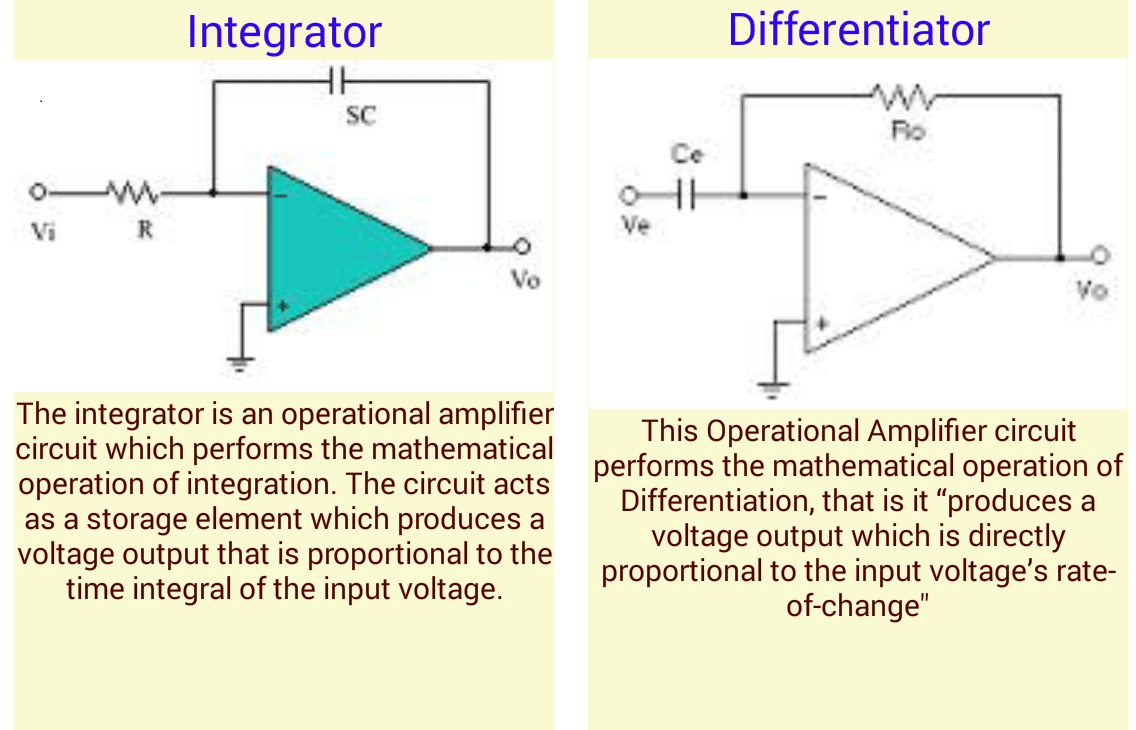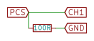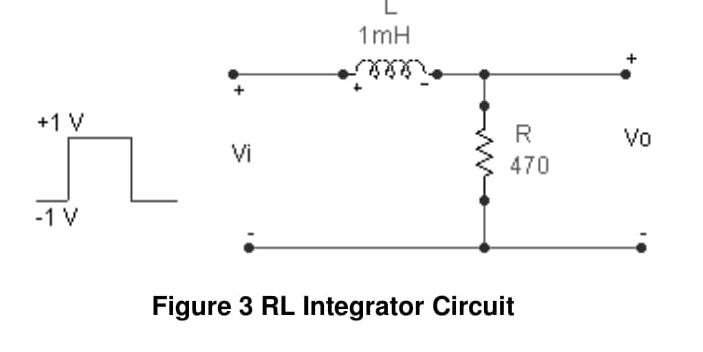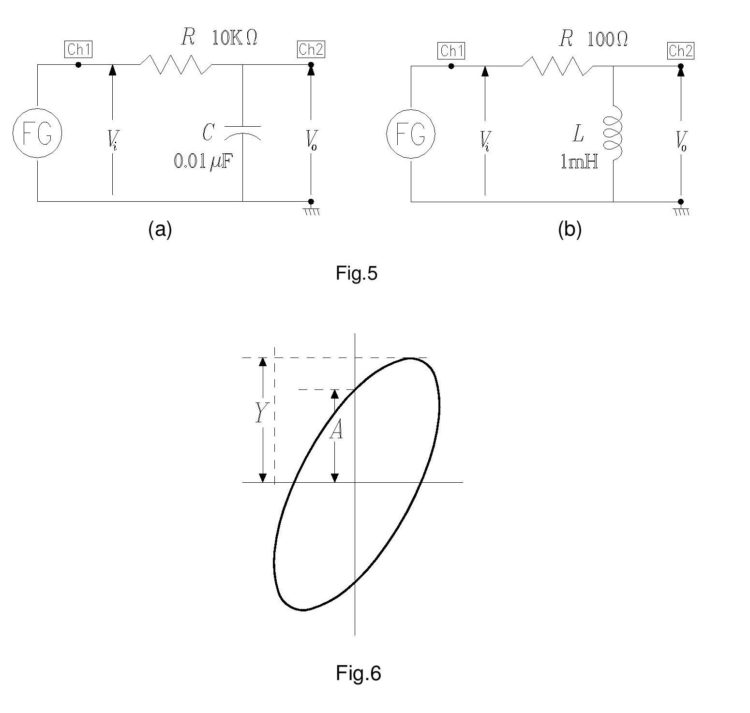# Electrical Experiments with PSLab

PSLab has the capability to perform a variety of experiments. The PSLab Android App and the PSLab Desktop App have built-in support for over 70 experiments which are commonly performed by students. In addition to that, it can be used in other experiments conveniently. This blog post is in continuation with the previous two posts regarding performing experiments (links in the reference) and this blog deals with another category of experiments that can be performed using PSLab.

The blog lists experiments which mainly involve the basic circuit elements like resistors, capacitors and inductors. These experiments involve the study of R-C, L-R, L-C and L-C-R circuits. These circuits have properties which make them important in real life applications and this blog attempts to give a rough picture of their importance.

Ohm’s Law, Capacitive Reactance and Inductive Reactance

These experiments involve the study of each of the basic circuit element individually. The current and voltage characteristics of each of the elements is studied.

The definitions of the above are:

Ohm’s Law – This is a law familiar to most. It relates the voltage and current of a purely resistive circuit stating that the voltage and current are proportional to each other and their ratio is a constant called the resistance. In this case, the current and voltage are in the same phase.

Capacitive Reactance – Across a capacitor in an AC circuit, the current and voltage are not in the same phase and the current leads the voltage. For a purely capacitive circuit, this difference is 90o.

Inductive Reactance –  Across an inductor in an AC circuit, the current and voltage are not in the same phase and the current lags behind the voltage. For a purely inductive circuit, this difference is 90o.

The reactance is given for capacitor and inductor is given by 1/wC and wL respectively, where C & L are the values of capacitance and inductance respectively and w is the frequency of the AC signal.

The circuit for the setup is shown below. We need to observe the plot of the input waveform and the plot of the voltage across individual elements to observe the phase shift.1. Connect CH1 & GND across the input terminals and CH2 & GND across the terminals of any of the elements.
2. An external signal can be used or can be generated using the PSLab. Use the PSLab to generate a sinusoidal signal of frequency 1000 Hz. by connecting the ends of PV1 in the circuit.
3. Observe the waveforms. In case of the resistor, there should be no observable phase lag between the two. In case of the capacitor and inductor, there will be an observable phase difference of 90o.
4. For the capacitive and inductive circuits, just replace the resistor in the above circuit with capacitor/inductor.

RC Circuits

Drawing their names from their respective calculus functions, the integrator produces a voltage output proportional to the product (multiplication) of the input voltage and time; and the differentiator (not to be confused with differential) produces a voltage output proportional to the input voltage’s rate of change.

RC Integrator circuit

For constructing the RC integrator circuit, connect the circuit as shown in the diagram.• Construction of the integrator circuit is fairly simple once the differentiator circuit is done.
• Interchange the positions of the capacitor and resistor in the above circuit and the circuit for the integrator is complete.
• Observe the output waveform. Plot both the CH1 and CH2 data simultaneously to compare the waveforms.

RC Differentiator circuit

For constructing the RC differentiator circuit, connect the circuit as shown in the diagram.• The values of resistance and capacitance used here are 10k ohm and 0.01uF.
• Connect the CH1 and GND pins of the board with the input side marked as Vi. Ensure that GND is connected to the GND of the circuit.
• Similarly, connect CH2 and GND with the corresponding ends of the output side marked as Vo.
• PSLab can also be used for supplying the input to the circuit. Connect the ends of W1 and GND across Vi. W1 can be used to generate a square wave of 10V peak to peak voltage with a frequency of 500 Hz.
• Observe the output waveform. Plot both the CH1 and CH2 data simultaneously to compare the waveforms.

RL Circuits

RL Integrator Circuit.

For constructing the RL integrator circuit, connect the circuit as shown in the diagram.• Construction of the integrator circuit is fairly simple once the differentiator circuit is done.
• Interchange the positions of the inductor and resistor in the above circuit and the circuit for the integrator is complete.
• Observe the output waveform. Plot both the CH1 and CH2 data simultaneously to compare the waveforms.

RL Differentiator Circuit

For constructing the RL differentiator circuit, connect the circuit as shown in the diagram.• The values of resistance and inductance used here are 470 ohm and 10mH.
• Connect the CH1 and GND pins of the board with the input side marked as Vi. Ensure that GND is connected to the GND of the circuit.
• Similarly, connect CH2 and GND with the corresponding ends of the output side marked as Vo.
• PSLab can also be used for supplying the input to the circuit. Connect the ends of W1 and GND across Vi. W1 can be used to generate a square wave of 2V peak to peak voltage with a frequency of 5000 Hz.
• Observe the output waveform. Plot both the CH1 and CH2 data simultaneously to compare the waveforms.

Frequency Response

Frequency Response of an electric or electronics circuit allows us to see exactly how the output gain (known as the magnitude response) and the phase (known as the phase response) changes at a particular single frequency, or over a whole range of different frequencies from 0Hz, (d.c.) to many thousands of megahertz, (MHz) depending upon the design characteristics of the circuit.

Frequency response of a circuit can be studied using different tools like Bode plots, phase plots etc. However, this blog would limit to using simple RC and RL circuits as they can be easily visualised using an oscilloscope.

RC Circuits

• For observing the frequency response of RC circuits, the circuit can be constructed as shown below.
• The values of resistance and capacitance used here are 10k ohm and 0.01uF.
• Connect the CH1 and GND pins of the board with the input side marked as Vi. Ensure that GND is connected to the GND of the circuit.
• Similarly, connect CH2 and GND with the corresponding ends of the output side marked as Vo.
• PSLab can also be used for supplying the input to the circuit. Connect the ends of W1 and GND across Vi. W1 can be used to generate a square wave of 10V peak to peak voltage with a frequencies ranging from 100 Hz to 5000 Hz.
• Switch to the X-Y mode of the oscilloscope and observe the waveform formed.RL Circuits

• For observing the frequency response of RL circuits, the circuit can be constructed as shown below.
• The values of resistance and inductance used here are 470 ohm and 10mH.
• Connect the CH1 and GND pins of the board with the input side marked as Vi. Ensure that GND is connected to the GND of the circuit.
• Similarly, connect CH2 and GND with the corresponding ends of the output side marked as Vo.
• Note: PSLab in this case cannot be used as an AC source as the maximum frequency of waveforms produced by PSLab is limited to 5kHz. However, this experiment would also need frequencies much higher than 5 Hz i.e upto 50 kHz. So, a dedicated function generator is needed. However, the oscilloscope would work just fine.
• Switch to the X-Y mode of the oscilloscope and observe the waveform formed.

References:

1. The previous blog on experiments using PSLab focusing in electronics https://blog.fossasia.org/electronics-experiments-with-pslab/
2. The previous blog on experiments using PSLab involving some general experiments https://blog.fossasia.org/fascinating-experiments-with-pslab/
3. Read more about differentiators and integrators and their uses https://www.allaboutcircuits.com/textbook/semiconductors/chpt-8/differentiator-integrator-circuits/